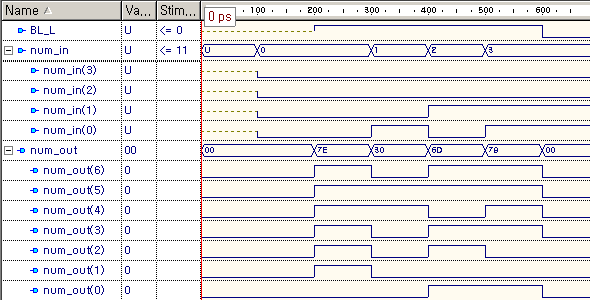# PGM for 74×49 (7 segments decoders)

<ABEL CODE>

module SSEGDEC
title ‘asdfas’
SEGDEC device ‘P22V10’;

“input pins
!BL pin 2;
D,C,B,A pin 3,4,5,6;
“output pins
Oa,Ob,Oc,Od,Oe,Of,Og pin 23,22,21,20,19,18,17;
Out = [Oa,Ob,Oc,Od,Oe,Of,Og];

equations
when (!BL==1) then Oa = (!A&!C)#(A&B&!D)#(A&C&!D)#(B&C&D)#(!A&D)#(!B&!C&D);
when (!BL==1) then Ob = (!C&!D)#(!A&!B&!D)#(A&B&!D)#(A&!B&D)#(!A&!C);
when (!BL==1) then Oc = (!B&!D)#(A&!D)#(A&!B)#(C&!D)#(!C&D);
when (!BL==1) then Od = (!A&!B&!C)#(A&!B&C)#(A&B&!C)#(A&B&!D)#(!A&C&D);
when (!BL==1) then Oe = (!A&!C)#(C&D)#(!A&B)#(B&D);
when (!BL==1) then Of = (!A&!B)#(!C&D)#(B&D)#(!B&C&!D)#(!A&C&!D);
when (!BL==1) then Og = (!A&B)#(A&D)#(!C&D)#(B&!C)#(!B&C&!D);
when (!BL==0) then Out = 0;
end SSEGDEC

<VHDL CODE>

library IEEE;
use IEEE.std_logic_1164.all;

entity segdec is
port ( num_in : in std_logic_vector(3 downto 0);
num_out : out std_logic_vector(6 downto 0);
BL_L : in std_logic );
end segdec;

architecture segdec_arch of segdec is
begin
process(num_in, BL_L)
begin
if  (BL_L) = ‘1’ then
case  num_in is
when “0000” => num_out <= “1111110”;
when “0001” => num_out <= “0110000”;
when “0010” => num_out <= “1101101”;
when “0011” => num_out <= “1111001”;
when “0100” => num_out <= “0110011”;
when “0101” => num_out <= “1011011”;
when “0110” => num_out <= “0011111”;
when “0111” => num_out <= “1110000”;
when “1000” => num_out <= “1111111”;
when “1001” => num_out <= “1110011”;
when “1010” => num_out <= “1110111”;
when “1011” => num_out <= “0011111”;
when “1100” => num_out <= “1001110”;
when “1101” => num_out <= “0111101”;
when “1110” => num_out <= “1001111”;
when “1111” => num_out <= “1000111”;
when others => num_out <= “0000000”;
end case;
else
num_out <= “0000000”;
end if;
end process;
end segdec_arch;